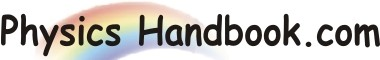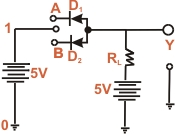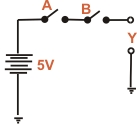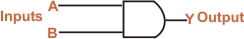HOME TOPICS DEFINITIONS TABLES LAWS INVENTIONS EXPERIMENTS QUIZ VIDEOS
 A B C D E F G H I J K L M N O P Q R S T U V W X Y Z
AND Gate
The AND gate is a logic gate that has two or more inputs but only one output. However, the output Y of an AND gates is HIGH when all inputs are HIGH. The output Y of an AND gate is LOW if any or all the inputs are LOW.
It is called AND gate because the output is high only when all the inputs are high. For this reason, the AND gate is sometimes called "any or nothing gate". For example, consider a 2-input AND gate. The output y will be high when both the input are high.Fig (a) Fig (b)
AND gate Operation : Fig (a) shown one way to build a 2-inputs AND gate while Fig (b) shows its simplified schematic diagram. There are only four input-output possibilities.
(I) When both A and B are connected to ground, both diodes (D1 and D2) are forward biased and hence they conduct current. Consequently, the two diodes are grounded and output voltage is zero. In terms of binary, when A = 0 and B = 0, then Y = 0 as shows in the truth table.

(II) When A is connected to ground and B connected to the positive terminal of the battery, diode D1 is forward biased and diode D2 will not conduct. Therefore diode D1 conducts and is grounded. Again output voltage will be zero. In terms of binary, When A = 0 and B = 1,then Y = 0 as shows in the truth table. (III) When both B is connected to the ground and A connected to the positive terminal of the battery, the roles of diodes are interchanged. Now diode D2 will conduct while diode D1 dose not conduct. As a result, diode D2 is grounded and again output voltage is zero. In binary terms when A = 1 and B = 0 then Y = 0 as shows in the truth table.

(IV) When both A and B are connected to the positive terminal of the battery, both diodes don't conduct. Now, the output voltage is +5V because there is no current through RL.
It is clear from the truth table that for AND gate, the output is high if all the input are high. However, the output is low if any or all input are low. Fig (c) shows the logic symbol of the AND gate. This is the symbol you will memories and use from now on for AND gates.
A
B
Y

0
0
0
0
1
0
1
0
0
1
1
1
Truth Table
Boolean Expression : The Boolean expression for AND function is

A . B = Y

where the multiplication " . (dot)" stands for the AND operation. The adjoining table shows the possible for the inputs. The table tells us 0 AND with any variable equals 0.1 AND with 1 equals one. The And function can be summed up as under:A . B
Y

0 . 0
0
0 . 1
0
1 . 0
0
1 . 1
1
0 AND with 0 equals 0
0 AND with 1 equals 0
1 AND with 1 equals 1
Fig (c)